# Multiplicative functions form abelian group under Dirichlet product

## Statement

Suppose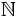is the set of natural numbers andis a commutative unital ring. Letbe the set of all multiplicative functions fromto, i.e., all the functions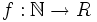satisfying the following:

•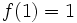,
•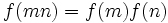wheneverare relatively prime.

Consider the Dirichlet product, a binary operation defined on functions fromto:.

Then,forms an abelian group under the Dirichlet product, with multiplicative identity given by the functionthat takes the valueatandelsewhere.

## Proof

### Closure under Dirichlet product

We first need to show that the Dirichlet product is a well-defined binary operation on multiplicative functions; in other words, that a Dirichlet product of multiplicative functions is multiplicative.

Given: Multiplicative functions.

To prove: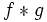is multiplicative.

Proof: Supposeandare relatively prime natural numbers. Consider:.

Given any divisorof,can be expressed uniquely as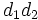, where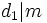and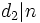. Conversely, givenand,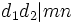. Thus, we have:.

Next, sinceandare relatively prime andand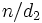are also relatively prime, andandare multiplicative: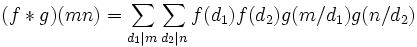.

Next, we rearrange: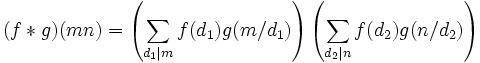.

Thus: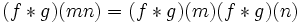.

### Associativity and commutativity

These follow from the fact that the Dirichlet product is associative and commutative for all functions:

### Identity element

This follows from the fact that the functionis an identity element for the Dirichlet product for all functions: Identity element for Dirichlet product is indicator function for one.

### Inverses

Given a multiplicative function, the inverse ofcan be defined inductively as follows:.

Since, this clearly satisfies: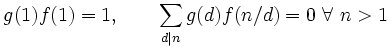.

Thus,. Since the multiplication is commutative,is a two-sided inverse for.

Next, we need to verify thatis multiplicative. Fill this in later